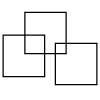#### You may also like### Three Squares

What is the greatest number of squares you can make by overlapping three squares?### Two Dice

Find all the numbers that can be made by adding the dots on two dice.### Biscuit Decorations

Andrew decorated 20 biscuits to take to a party. He lined them up and put icing on every second biscuit and different decorations on other biscuits. How many biscuits weren't decorated?

# Sort Them Out (1)

##### Age 5 to 7 Challenge Level:

This problem provided a good opportunity to work with each other as a team. The cards gave you some practice with addition and subtraction, as well as challenging you to consider different ways of arranging them.

Courtenay and Emily from Ickford Combined School explained their method of sorting the cards:

We sorted the answers out in the two times table and the four times table and the ones that are in both times table and another group which is not in either the two and four times table.

Remember that all numbers in the four times table will feature in the two times table.

Caroline from Estes Hills Elementary, North Carolina, USA (Hello to the USA!) submitted her answer:

At first I sorted ten by itself and the teens together and the twentys together. Then I noticed that I could sort them by number order from $10$ to $24$.

Matthew from Stambridge looked at odd and even numbers:

First we separated the cards into additions and subtractions.
Next we looked at the answers.
In the addition pile we saw that:
E+E=E
O+O=E
E+O=O
O+E=O
In the subtraction pile we saw that:
E-O=O
O-E=O
O-O=E
E-E=E
So, you get an even answer from:
E-E
E+E
O+O
O-O
And so you get an odd answer from:
E-O
E+O
O-E
O+E

Can you think of other ways of arranging the cards?

Now have a go at Sort Them Out (2).